# Digging Deeper into … Decimals and Percentages (3rd – 6th)

## Digging Deeper into … Decimals and Percentages (3rd – 6th)

Category : Uncategorized

For practical suggestions for families, and links to useful digital resources, to support children learning about this topic, please check out the following post: Dear Family, your Operation Maths Guide to Decimals and Percentages

In Operation Maths, decimals sits as a chapter of its own in third and fourth classes (allowing for specific time to focus on this new concept) and as a chapter combined with fractions and percentages in 5th and 6th classes. However, it is worth noting that the children would have informally encountered decimals since being introduced to euro and cent in first class. And, since decimals are inherently linked with both fractions and the place value system*, these topics, also, were encountered initially in first class, and thus the children’s understanding of decimals and percentages in the senior classes builds on their understanding of these related concepts. Indeed, in Operation Maths 5 and 6, the place value chapters include both whole and decimal numbers, since both are part of our base-ten system.

HINT: Read the related Operation Maths blog posts on fractions and place value at the links given.

### Representing decimals and percentages

In keeping with the CPA approach used throughout Operation Maths, the initial introductory activities concentrate on experiences with concrete materials (e.g. straws and money, as shown below) and pictorial activities (e.g. colouring in fractions of shapes, dividing shapes and completing number lines) before progressing to abstract questions, where the focus is primarily on numbers and/or digits.  Concrete and pictorial-based experiences also promote the development of number sense and visualisation skills, allowing the children to become more adept at converting between various forms and, ultimately, being able to order, compare and calculate with fractions, decimals and percentages more efficiently and accurately.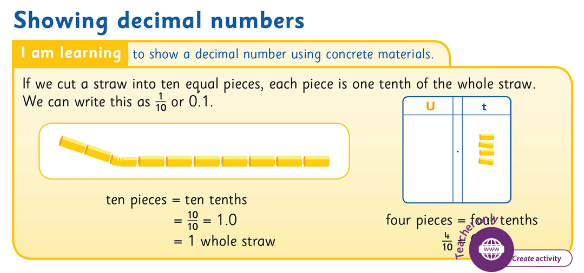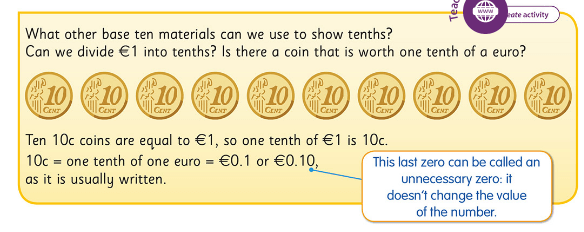In third class, the children encounter straws, place value discs, euro coins and blocks as examples of base-ten materials to represent hundreds, tens and units. Since, to represent one tenth, it is necessary to be able to fraction a unit, the only suitable base-materials to use are straws and ten cent coins (ie one tenth of a euro). Cutting up a straw into tenths really helps to demonstrate that one tenth is a very small part or bit of the whole. Operation Maths users can also use the Place Value eManipulative, accessible via Edco Learning to show various decimal numbers on the class IWB (see below).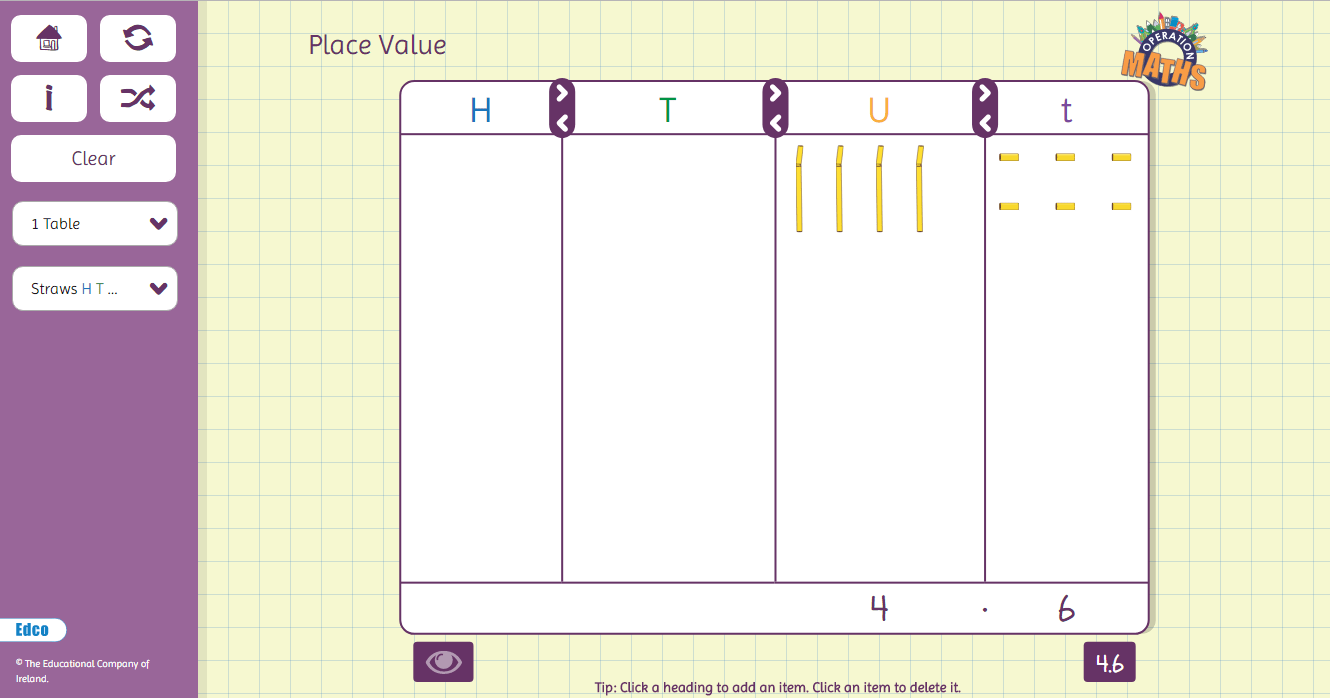In fourth class, with the introduction of the hundredth, the concrete materials become more limited, and one cent coins are used to represent hundredths of a euro (see below). Again, the Place Value eManipulative can also be used to represent hundredths; just select the Money HTU.th option from the drop down menu.NB: With rounding of prices to the nearest 5c, we are likely to see fewer 1c and 2c coins in circulation, although recognition and knowledge of 1c and 2c coins are still part of the curriculum. Therefore, it was decided to continue to include them throughout the Operation Maths series for both this reason and because they are valuable for teaching purposes, especially when teaching decimals, as shown above.

By fifth class, with the introduction of thousandths, the concrete possibilities have become even more limited; there is no coin to represent one thousandth of a euro because it is such a small and insignificant amount.  To this end, it it necessary to re-use base ten blocks, but with new values assigned to each block type (see below). It is very important that this is emphasized to the children,  and that they understand that the block that was previously used to represent a unit in 3rd and 4th class, is now being used to represent a thousandth in 5th and 6th class, simply because there are no other options!Types of traditional representations for decimal numbers that have deliberately not been used throughout the Operation Maths series are place value abaci and dot notation boards. As mentioned previously in the post on Place Value, tasks which just involve the children identifying the number of dots on a notation board, or the number of beads on a place value abacus have not been included as they are not good indicators of a child’s understanding; rather they are simply demonstrating their number knowledge of numbers and digits from 0–9.

With the introduction of percentages in fifth class, since percentages are directly related to hundredths, all of the concrete resources that can represent tenths and hundredths can be used again to represent a percentage. When exploring how percentages relate to fractions and decimals, multiple hundredths squares can be used to encourage the visualisation of the various fractions, as in done in the Operation Maths Discovery book for fifth class (see below).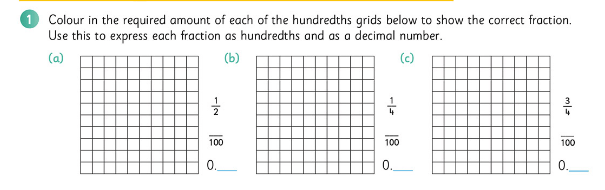### ‘Same value, different appearance’

Because of the close connections between decimals, percentages and fractions, it is very important from the beginning that decimals (or to be more exact, decimal fractions) and percentages (from their introduction in 5th class), are taught as connected concepts with fractions (e.g. 1/2 = 0.5 = 50%,  1/4 = 0.25 = 25%) and that the children are encouraged to recognise them as different forms of an equivalent value i.e. ‘same value, different appearance’. Being able to convert into equivalent forms becomes very important when it comes to ordering parts of a whole that are expressed in various forms (see below).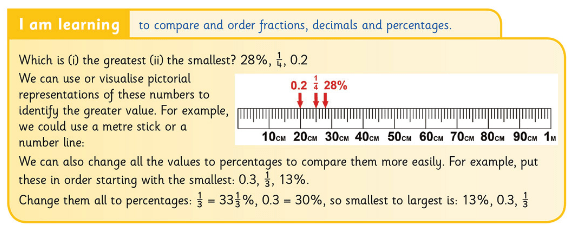Similarly, the children should be encouraged from the beginning to use both decimal language and fractional language when verbalising decimal notation, i.e. expressing 7.38 as ‘seven point three eight’ and also as ‘seven and thirty-eight hundredths’*. Using fractional language to read decimals reinforces the value of the digit(s) in the decimal place(s).

*However, when using decimal language, it is mathematically incorrect to say ‘seven point thirty-eight’, as the suffix -ty means tens.

Furthermore, the same decimal value can be written in various ways eg one-tenth can be written as 0.1, .1, 0.10, 0.100, etc. Many teachers often use only one form, usually 0.1, fearing that a variety of ways may confuse children. Conversely, using a variety of ways can actually help reinforce children’s understanding that all the above forms show one-tenth, with all forms (excluding .1) including unnecessary zeros.*
*Zeros can be necessary or unnecessary. In 30, the zero is necessary as without it, the value would be 3 units. In 0.3, the zero is unnecessary as without it, the value is still three-tenths.

### Calculations with decimals and percentages

Addition and subtraction involving decimals can often appear to be mastered, until the children start using decimals of differing lengths (sometimes referred to as ragged decimals), and subsequent errors at this stage can reveal gaps in the children’s understanding of the concept. Remind the children regularly to lay out calculations so that the decimal places and decimal points are in line, and also use concrete materials (e.g. €1, 10c and 1c coins shown earlier) to encourage them to visualise the component parts of the number and how these similar parts must be added or subtracted accordingly e.g. for 1.24 + 2.3,  ‘4 hundredths and zero hundredths equals 4 hundredths, 2 tenths and 3 tenths equals 5 tenths’ etc.

Similarly, multiplication with decimals can also reveal gaps in understanding e.g. 0.4 × 3 might be answered as 0.12; again, where possible use concrete materials (e.g. use the tenths of the straws) to encourage the children to visualise the numbers i.e. ‘4 tenths times 3  (or three groups of four tenths) is 4 tenths plus 4 tenths plus 4 tenths, which is 12 tenths’; if straws are used we can see how that is equal to one whole straw and 2 tenths i.e. 1.2. Therefore, if interpreting multiplication as “group(s) of”, it is important that the children appreciate that 0.5 x 3 can be thought of as 1/2 group of 3 or as 3 groups of 1/2, both of which equal 1 1/2; this is modeled below using the Operation Maths Bar Model eManipulative, accessible on edcolearning.ie.Another way to think about multiplications is as “rows of” and this concept of row leads very logically to the area model of multiplication. Base ten blocks are a very useful concrete material that can be used to represent multiplication with decimals via the area model, however it is important that the children appreciate that we are giving new values to each block ie each flat represents 1, the rods each represent 0.1/one tenth and the small cubes each represent 0.01/one hundredth. For more on how to use the area model of multiplications with decimals, please read on here: https://www.mathcoachscorner.com/2015/09/multiplying-decimals/The cartoon above clearly illustrates how fostering number sense is as important as teaching procedures:
2.95 is nearly 3
So 2.95 x 3.2 is roughly 3 x 3 which is 9.something
The decimal point therefore goes in after 9

When solving a problem involving percentages, it is generally more efficient to convert the percentage to an equivalent fraction or decimal, and then solve the problem using a fraction or decimal approach; the decimal approach is often the most efficient  to use in a situation where a calculator is available/allowed. When using a fraction approach the children should be encouraged to use bar models to represent the quantities involved (see below).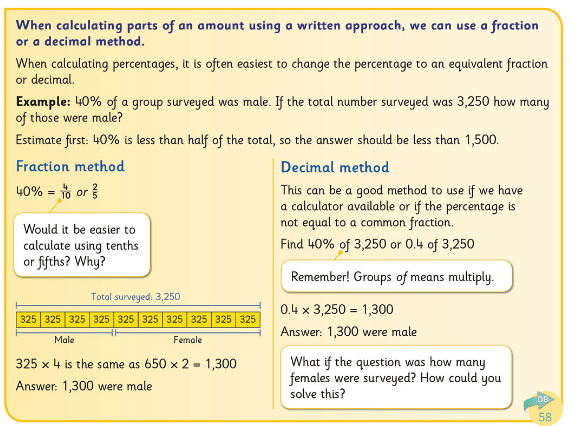All activities should emphasise understanding and not just a procedural approach.
While it is important that children in sixth class are shown ways to calculate with more complex numbers, it is vital that this is done in such a way that the children begin to understand the purpose of the approaches and how they work (see below). Being told to just ‘multiply by 100/1’ or ‘divide by the bottom, multiply by the top’, does little to enable the child to understand the concept better.### Incorrect assumptions & misconceptions

As discussed earlier, while fractions, decimals, percentages and place value understanding are inherently connected, children may apply whole number and fractional understanding to decimals and percentages in such a way as to make incorrect assumptions.

For example:

• A child may incorrectly assume that a number with more digits is bigger than a number with fewer digits, i.e. assuming incorrectly that 2.1 < 1.35
• In fractions, as the denominator increases the fraction parts themselves get smaller (e.g. 1/2 > 1/10). Therefore, a child may assume, also incorrectly, that a decimal number with more digits is smaller, e.g. 1.32 < 1.2.
• If 1/4 is greater than 1/8 , then a child may assume that 1.4 is greater than 1.8.
• A child may convert a decimal value directly to a percentage (and vice versa), without changing their value, e.g. incorrectly assuming 0.15 = 0.15%.

There can be many other commonly held misconceptions and errors, including a child incorrectly thinking that:

• Fractions, decimals and percentages are only parts of shapes and not numbers in their own right.
• Percentages don’t go above 100% and fractions are never greater than 1.
• A fraction such as 3/4 is only 3 groups of 1/4 without recognition that it can also be a 1/4  of 3; this is essential to understanding how a fraction can be converted into a decimal.
• To calculate 20% of an amount, you divide by 20 (since to calculate 10% you divide by 10).
• 0.2 equals a half or 12% is one twelfth, etc.

Again, many of these misconceptions and incorrect assumptions can be avoided by using a CPA approach to the teaching of this topic, with an emphasis on understanding rather than just doing.